Related Articles

# PySpark DataFrame – Select all except one or a set of columns

• Last Updated : 17 Jun, 2021

In this article, we are going to extract all columns except a set of columns or one column from Pyspark dataframe. For this, we will use the select(), drop() functions.

But first, let’s create Dataframe for demonestration.

## Python3

 `# importing module``import` `pyspark`` ` `# importing sparksession from pyspark.sql module``from` `pyspark.sql ``import` `SparkSession`` ` `# creating sparksession and giving an app name``spark ``=` `SparkSession.builder.appName(``'sparkdf'``).getOrCreate()`` ` `# list  of students  data``data ``=` `[[``"1"``, ``"sravan"``, ``"vignan"``],``        ``[``"2"``, ``"ojaswi"``, ``"vvit"``],``        ``[``"3"``, ``"rohith"``, ``"vvit"``],``        ``[``"4"``, ``"sridevi"``, ``"vignan"``],``        ``[``"1"``, ``"sravan"``, ``"vignan"``],``        ``[``"5"``, ``"gnanesh"``, ``"iit"``]]`` ` `# specify column names``columns ``=` `[``'student ID'``, ``'student NAME'``, ``'college'``]`` ` `# creating a dataframe from the lists of data``dataframe ``=` `spark.createDataFrame(data, columns)`` ` `print``(``'Actual data in dataframe'``)``dataframe.show()`

Output: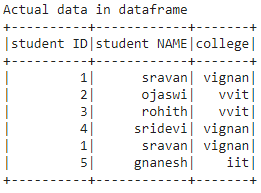### Method 1: Using drop() function

drop() is used to drop the columns from the dataframe.

Syntax: dataframe.drop(‘column_names’)

Where dataframe is the input dataframe and column names are the columns to be dropped

Example: Python program to select data by dropping one column

## Python3

 `# drop student id``dataframe.drop(``'student ID'``).show()`

Output: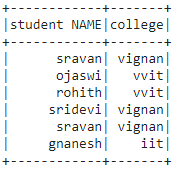Example 2: Python program to drop more than one column(set of columns)

## Python3

 `# drop student id and college``dataframe.drop(``'student ID'``,``'college'``).show()`

Output: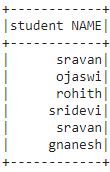### Method 2: Using select() function

This function is used to select the columns from the dataframe

Syntax: dataframe.select(columns)

Where dataframe is the input dataframe and columns are the input columns

Example 1: Select one column from the dataframe.

## Python3

 `# select student id ``dataframe.select(``'student ID'``).show()`

Output: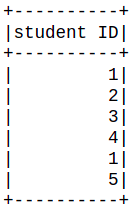Example 2: Python program to select two columns id and name

## Python3

 `# select student id and student name``dataframe.select(``'student ID'``,``'student NAME'``).show()`

Output: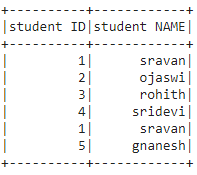Attention geek! Strengthen your foundations with the Python Programming Foundation Course and learn the basics.

To begin with, your interview preparations Enhance your Data Structures concepts with the Python DS Course. And to begin with your Machine Learning Journey, join the Machine Learning – Basic Level Course

My Personal Notes arrow_drop_up